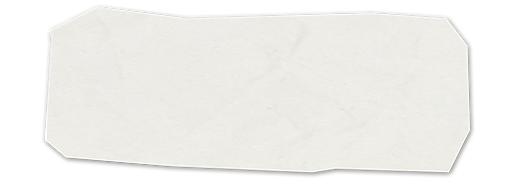## 9. Stoichiometry

9.1 Introduction to stoichiometry.  Additional practice problems can be found in the Holt Modern Chemistry textbook.  Try practice problems A-D on pages 289-293, modeling and answers are provided.

Work shop 9.1 steps and answers!

9.2 stoichiometry conversions for mass to mass, and molecules to mass.  Additional practice problems can be found in the Holt Modern Chemistry textbook.  Try practice problems E on pages 294-295, modeling and answers are provided.

Work shop 9.2 steps and answers!

9.3 Limiting reactants and percent yield.  Additional practice problems can be found in the Holt Modern Chemistry textbook.  Try practice problems F-H on pages 296-302, modeling and answers are provided.

Work shop 9.3 steps and answers!

Stoichiometry practice problem [Solved]